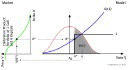Apr 2006

# Equity Markov Functional ModelI wrote a small note on how to do Markov Functional Modeling for a single asset (like equity). The "trick" only works if you use the asset itself as Numéraire. However, on the other hand, this is exactly what you do in Markov Functional Modeling of interest rates, where the Numéraire is an interest rate product. The approach in this note is very similar to the Markov Functional Model in spot LIBOR measure (the original Makov Functional Model was specified in terminal measure).
Version 0.4 of the paper is still lacking an introduction.

(Edit 14.04.06): In Version 0.8 I have added a nice discussion on model dynamics, using Black-Scholes like functionals as a starting point for my examples. The discussion shows how to calibrate the joint asset-interest rate dynamics (ie. r(S)) and forward volatility (all this in addition to the calibration to a full two dimensional smile surface.